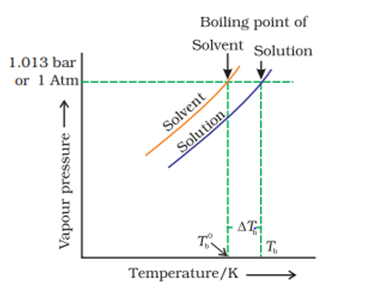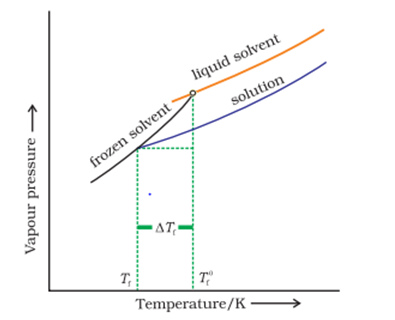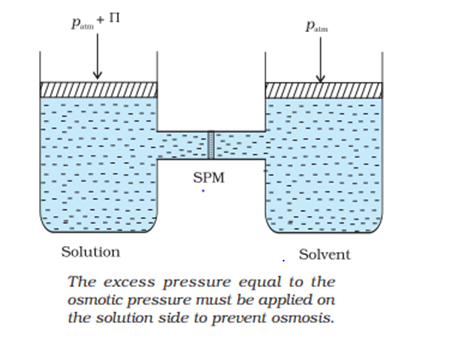# COLLIGATIVE PROPERTY

## COLLIGATIVE PROPERTY

These are the properties of a soln that depends upon the no. Of solute particle & do not depend upon nature of solute particle.
There are four colligative properties:-
1. Relative lowering in vapour pressure
2. Elevation in B. P.
3. Depression in freezing point
4. Osmotic pressure.
Condition:
1. The solute must be non- volatile.
2. The soln must be dilute.(no. of moles of solute particle << no. of moles of solvent particles)
3. The solute must not undergo any association or dissociation in the soln.

### Relative Lowering in vapour pressure

As per Raoult laws,
$P_A = P_A^O \chi _A$
Lowering vapour pressure = $P_A^O- P_A$
Relative lowering in vapour pressure = $\frac {(P_A^O- P_S )}{(P_A^O )}$
To show relative lowering in vapour pressure is a colligative property
According to Roault’s law for a soln containing non - volatile solute.
$P_S = P_A = P_A^O. \chi _A$
$P_S = P_A^O. \chi _A$
$P_S = P_A^O (1- \chi _B )$
$P_S = P_A^O.- P_A^O. \chi _B$
$P_S - P_A^O = - P_A^O. \chi _B$
$P_A^O- P_S = P_A^O. \chi _B$
$(P_A^O- P_S )/(P_A^O ) = \chi _B$
Relative vapour pressure=Mole fraction of solute
Relative vapour pressure depends upon no. of solute particles. Hence, it is a colligative property.

### Elevation in boiling point:-

The boiling point of a liquid is a temperature at which at vapour pressure becomes equal to atm. pressure (1 atm).
$\text {B. P.} \alpha \frac {1}{\text{(V.P.)}}$
The boiling point of soln ($T_b$) is greater than the boiling point of pure solvent ($T_b^o$)
Elevation in boiling point ($\Delta T_b$) = $T_b - T_b^o$
$\Delta T_b= T_b – T_b^o$
Experimentally, $\Delta T_b \alpha m$
$\Delta T_b= K_b.m$
$\Delta T_b= K_(b) \times \frac {(W_B \times 1000)}{(M_B \times W_A )}$
Where $K_b$ -- Molal elevation constant or ebullioscopic constant
$\Delta T_b= K_b \times m$
$K_b = \frac {\Delta T_b}{m}$
If, molarity = 1,$K_b = \Delta T_b$
Molal elevation constant of a solvent is equal to elevation in boiling point of a soln of unit molality or 1 molal solution.
Unit – K kg/mol
If two different solutions have same solvent then the value of $K_b$ will be the same for both the solution.
Numerial value of $\Delta T_b$ will be same both in Celsius & Kelvin.
Ex: $\Delta T_b = 2^o C$
$\Delta T_b = 2$ K
Boiling point of H2O = $100^o C$ or 373.15 K### Depression in freezing point:-The freezing point of a substance is the temperature at which the vapour point of solid state is equal to the vapour pressure of its liquid state.
The freezing point of pure solvent ($T_f^o$) is greater than freezing point of soln ($T_f$).
Depression in freezing point ($\Delta T_f$)= $T_f^o- T_f$
Experimentally, $\Delta T_f \alpha m$
$\Delta T_f= K_f.m$
$K_f$ ->Molal depression constant or cryoscopic constant
$\Delta T_f= K_f \times \frac { (W_B \times 1000)}{(M_B \times W_A )}$
$K_f= \frac {\Delta T_f}{m}$
If molality = 1, $K_f= \Delta T_f$
Molal depression constant for a solvent is equal depression in freezing point of a molal solution.
Unit is K kg/mol
Freezing point of water: $0^o C$ or 273.15 K

### Anti Freezing Mixture:-

Anti – freezing mixture is a mixture in which the freezing point of water is less than 0o C. The freezing point of water can be decreased by adding a non – volatile solute to it.
For eg: Mixture of Ethelene glycol & water is an anti freeze mixture & the freezing point of water can be lowered to as low as – 42o C.

### Osmosis and Osmotic Pressure

When two liquids of different concentrations are separated by a semi – permeable membrane then solvent particles move from lower conc. to higher concentration and this process is called Osmosis.

Osmotic pressure:Osmotic pressure of a solution is equal to the external pressure applied on higher conc. side so as to just stop the process of osmosis. [External pressure = $\prod$ ]
Factors affecting osmotic pressure:-
1. $\prod \alpha C$ [Depends upon concentration]
2. $\prod \alpha T$ [Depends upon temperature]
$\prod \alpha C. T$
$\prod = C. R. T$
So,
$\prod= \frac {(W_B \times 1000)/}{(M_B \times V_S )} \times R \times T$
When $\prod$ is in atm. then R = 0.0821 L atm /K.mol
& $\prod$ is in bar then R = 0.083 L bar/K.mol
Reverse Osmosis [External pressure > $\prod$ ]
When external pressure is greater than the osmotic pressure then the solvent particles move from higher conc. to lower conc. through a semi = permeable membrane. This process is called reverse osmosis.
RO is a non = spontaneous process. We can use this process for Desalination of Sea water.
Endo Osmosis and Exo Osmosis
In Endo osmosis the substance swells & in Exo – osmosis the substance shrinks.
Hypertonic, Hypotonic and Isotonic solutions:-
The solution having higher osmotic pressure is termed as hypertonic solution and the other solution having lower osmotic pressure is termed as hypotonic solution.
For eg:- $\prod_A=3$ atm-→Hypotonic
$\prod_B=5$ atm-→Hypertonic
Solutions having same osmotic pressure are called isotonic solutions.